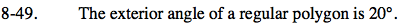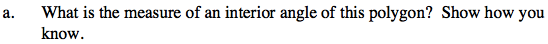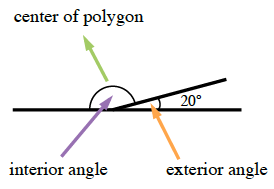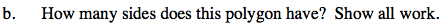Home > GC > Chapter 8 > Lesson 8.1.5 > Problem8-49

8-49.
1. The exterior angle of a regular polygon is 20°. Homework Help ✎

1. What is the measure of an interior angle of this polygon? Show how you know.

2. How many sides does this polygon have? Show all work.The interior and exterior angles are supplementary.Refer to the Math Notes box in Lesson 8.1.4.

Use one of the formulas to find the number of sides of the polygon.

Since we know the measure of the interior and exterior angles, the number of sides can be found in two ways.

$\text{number of sides}(n)=360\degree\div (\angle \text{exterior angle})$

$\text{Or, }\angle \text{interior angle}=\frac{180\degree(n-2)}{n}$

18